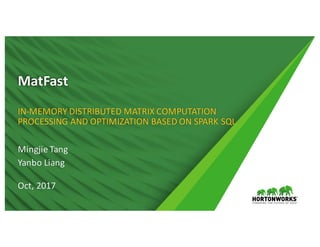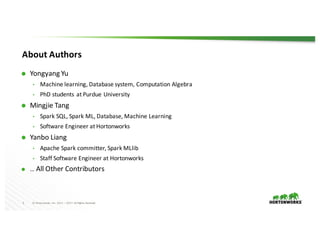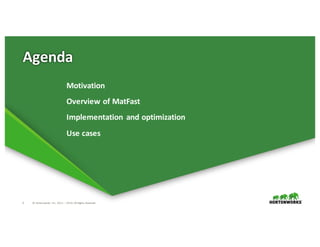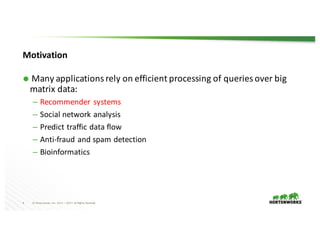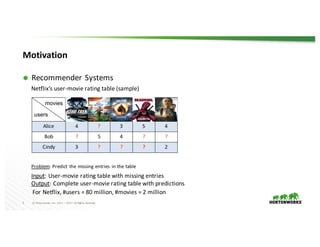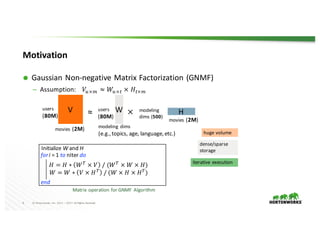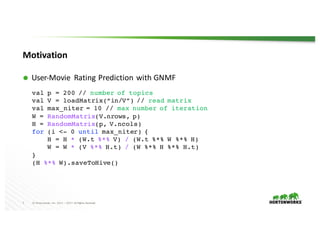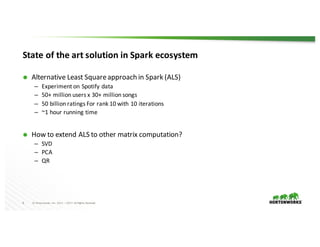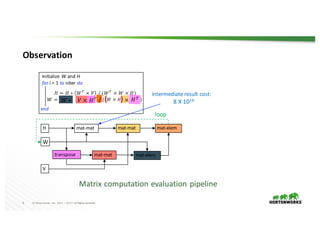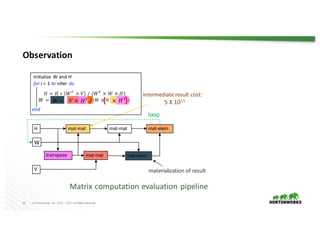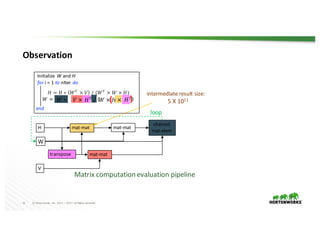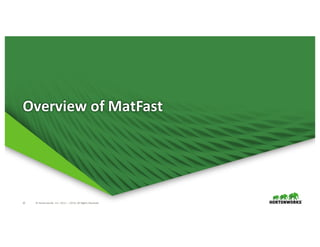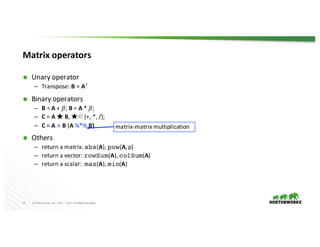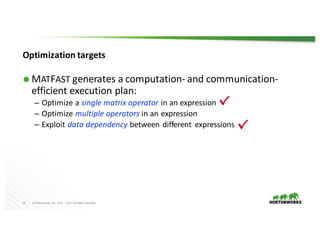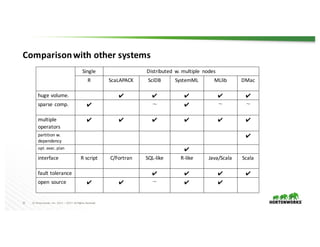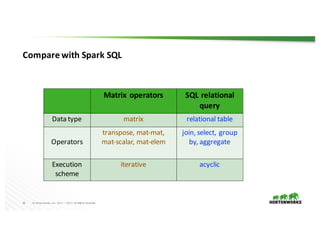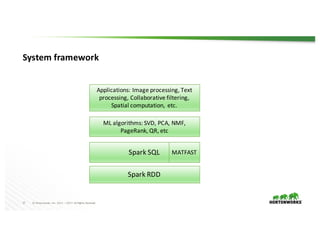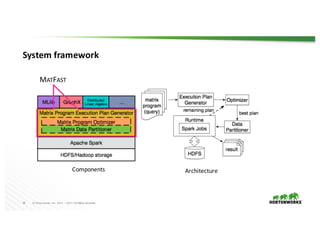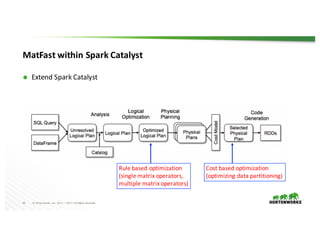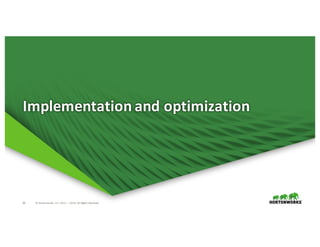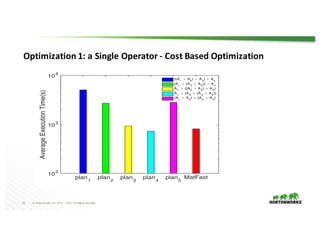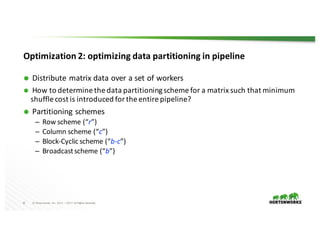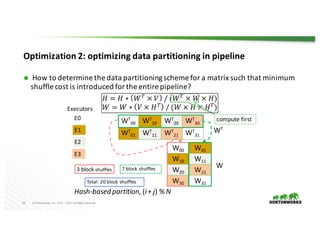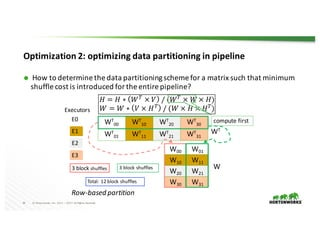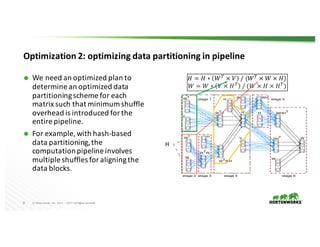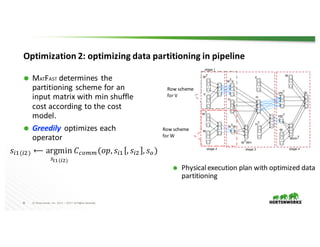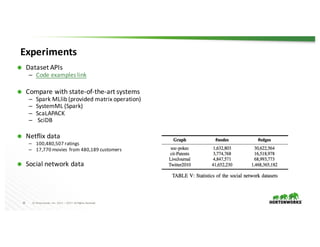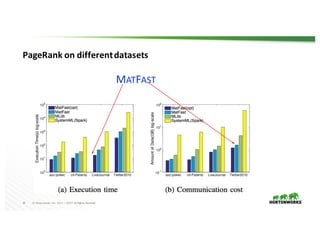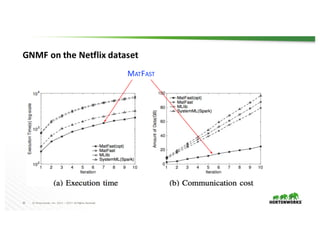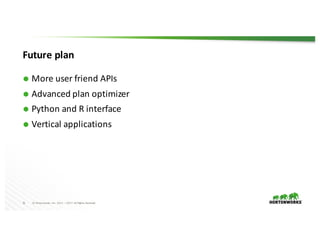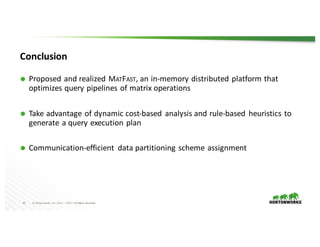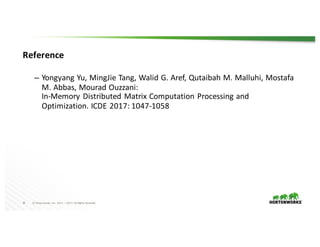1 of 34

### MatFast: In-Memory Distributed Matrix Computation Processing and Optimization Based on Spark SQL Yanbo Liang and Mingie Tang

1. 1 © Hortonworks Inc. 2011 – 2017. All Rights Reserved MatFast IN-MEMORY DISTRIBUTED MATRIX COMPUTATION PROCESSING AND OPTIMIZATION BASED ON SPARK SQL Mingjie Tang Yanbo Liang Oct, 2017
2. 2 © Hortonworks Inc. 2011 – 2017. All Rights Reserved About Authors Ã Yongyang Yu • Machine learning, Database system, Computation Algebra • PhD students at Purdue University Ã Mingjie Tang • Spark SQL, Spark ML, Database, Machine Learning • Software Engineer at Hortonworks Ã Yanbo Liang • Apache Spark committer, Spark MLlib • Staff Software Engineer at Hortonworks Ã … All Other Contributors
4. 4 © Hortonworks Inc. 2011 – 2017. All Rights Reserved Motivation Ã Many applications rely on efficient processing of queries over big matrix data: – Recommender systems – Social network analysis – Predict traffic data flow – Anti-fraud and spam detection – Bioinformatics
5. 5 © Hortonworks Inc. 2011 – 2017. All Rights Reserved Motivation Ã Recommender Systems Netflix’s user-movie rating table (sample) Problem: Predict the missing entries in the table Input: User-movie rating table with missing entries Output: Complete user-movie rating table with predictions For Netflix, #users = 80 million, #movies = 2 million Batma n begins Alice in Wonde rland Doctor Strange Trolls Ironma n Alice 4 ? 3 5 4 Bob ? 5 4 ? ? Cindy 3 ? ? ? 2 movies users
6. 6 © Hortonworks Inc. 2011 – 2017. All Rights Reserved Motivation Ã Gaussian Non-negative Matrix Factorization (GNMF) – Assumption: 𝑉"×\$ ≈ 𝑊"×' × 𝐻'×\$ V ≈ W H×users (80M) movies (2M) users (80M) modeling dims (e.g., topics, age, language, etc.) modeling dims (500) movies (2M) 𝐻 = 𝐻 ∗ 𝑊, × 𝑉 / (𝑊, × 𝑊 × 𝐻) 𝑊 = 𝑊 ∗ 𝑉 × 𝐻, / (𝑊 × 𝐻 × 𝐻, ) fori = 1 to nIter do end Initialize W and H Matrix operation for GNMF Algorithm huge volume dense/sparse storage iterative execution
7. 7 © Hortonworks Inc. 2011 – 2017. All Rights Reserved Motivation Ã User-Movie Rating Prediction with GNMF val p = 200 // number of topics val V = loadMatrix(“in/V”) // read matrix val max_niter = 10 // max number of iteration W = RandomMatrix(V.nrows, p) H = RandomMatrix(p, V.ncols) for (i <- 0 until max_niter) { H = H * (W.t %*% V) / (W.t %*% W %*% H) W = W * (V %*% H.t) / (W %*% H %*% H.t) } (H %*% W).saveToHive()
8. 8 © Hortonworks Inc. 2011 – 2017. All Rights Reserved State of the art solution in Spark ecosystem Ã Alternative Least Square approach in Spark (ALS) – Experiment on Spotify data – 50+ million users x 30+ million songs – 50 billion ratings For rank 10 with 10 iterations – ~1 hour running time Ã How to extend ALS to other matrix computation? – SVD – PCA – QR
9. 9 © Hortonworks Inc. 2011 – 2017. All Rights Reserved Observation H W transpose V mat-mat mat-mat mat-mat mat-elem mat-elem loop 𝐻 = 𝐻 ∗ 𝑊, × 𝑉 / (𝑊, × 𝑊 × 𝐻) 𝑊 = ( ) ( ) for i = 1 to nIter do end Initialize W and H Matrix computation evaluation pipeline 𝑊 ∗ 𝑉 × 𝐻, / 𝑊 × 𝐻 × intermediate result cost: 8 X 1016( )
10. 10 © Hortonworks Inc. 2011 – 2017. All Rights Reserved 𝑊 × 𝐻 Observation H W transpose V mat-mat mat-mat mat-mat mat-elem mat-elem intermediate result cost: 5 X 1011 loop materialization of result 𝐻 = 𝐻 ∗ 𝑊, × 𝑉 / (𝑊, × 𝑊 × 𝐻) 𝑊 = ( ) ( ) for i = 1 to nIter do end Initialize W and H Matrix computation evaluation pipeline 𝑊 ∗ 𝑉 × 𝐻, / 𝐻, )×(
11. 11 © Hortonworks Inc. 2011 – 2017. All Rights Reserved 𝑊 × 𝐻 Observation H W transpose V mat-mat mat-mat mat-mat chained mat-elem intermediate result size: 5 X 1011 loop 𝐻 = 𝐻 ∗ 𝑊, × 𝑉 / (𝑊, × 𝑊 × 𝐻) 𝑊 = ( ) ( ) for i = 1 to nIter do end Initialize W and H Matrix computation evaluation pipeline 𝑊 ∗ 𝑉 × 𝐻, / 𝐻,×( )
13. 13 © Hortonworks Inc. 2011 – 2017. All Rights Reserved Matrix operators Ã Unary operator – Transpose: B = AT Ã Binary operators – B = A + 𝛽; B = A * 𝛽; – C = A ★ B, ★∈{+, *, /}; – C = A B (A %*% B) Ã Others – return a matrix: abs(A), pow(A, p) – return a vector: rowSum(A), colSum(A) – return a scalar: max(A), min(A) matrix-matrix multiplication
14. 14 © Hortonworks Inc. 2011 – 2017. All Rights Reserved Optimization targets Ã MATFAST generates a computation- and communication- efficient execution plan: – Optimize a single matrix operator in an expression – Optimize multiple operators in an expression – Exploit data dependency between different expressions
15. 15 © Hortonworks Inc. 2011 – 2017. All Rights Reserved Comparison with other systems Single Distributed w. multiple nodes R ScaLAPACK SciDB SystemML MLlib DMac huge volume. ✔ ✔ ✔ ✔ ✔ sparse comp. ✔ 〜 ✔ 〜 〜 multiple operators ✔ ✔ ✔ ✔ ✔ ✔ partition w. dependency ✔ opt. exec. plan ✔ interface R script C/Fortran SQL-like R-like Java/Scala Scala fault tolerance ✔ ✔ ✔ ✔ open source ✔ ✔ 〜 ✔ ✔
16. 16 © Hortonworks Inc. 2011 – 2017. All Rights Reserved Compare with Spark SQL Matrix operators SQL relational query Data type matrix relational table Operators transpose, mat-mat, mat-scalar, mat-elem join, select, group by, aggregate Execution scheme iterative acyclic
17. 17 © Hortonworks Inc. 2011 – 2017. All Rights Reserved Spark SQL System framework MATFAST ML algorithms: SVD, PCA, NMF, PageRank, QR, etc Spark RDD Applications: Image processing, Text processing, Collaborative filtering, Spatial computation, etc.
19. 19 © Hortonworks Inc. 2011 – 2017. All Rights Reserved MatFast within Spark Catalyst Ã Extend Spark Catalyst Rule based optimization (single matrix operators, multiple matrix operators) Cost based optimization (optimizing data partitioning)
21. 21 © Hortonworks Inc. 2011 – 2017. All Rights Reserved Optimization 1: a Single Operator - Cost Based Optimization plan 1 plan 2 plan 3 plan 4 plan 5 MatFast AverageExecutionTime(s) 102 10 3 10 4 ((A1 × A2 ) × A3 ) × A4 (A1 × (A2 × A3 )) × A4 A1 × ((A2 × A3 ) × A4 ) A1 × (A2 × (A3 × A4 )) (A1 × A2 ) × (A3 × A4 )
22. 22 © Hortonworks Inc. 2011 – 2017. All Rights Reserved Optimization 2: optimizing data partitioning in pipeline Ã Distribute matrix data over a set of workers Ã How to determine the data partitioning scheme for a matrix such that minimum shuffle cost is introduced for the entire pipeline? Ã Partitioning schemes – Row scheme (“r”) – Column scheme (“c”) – Block-Cyclic scheme (“b-c”) – Broadcast scheme (“b”)
23. 23 © Hortonworks Inc. 2011 – 2017. All Rights Reserved Optimization 2: optimizing data partitioning in pipeline Ã How to determine the data partitioning scheme for a matrix such that minimum shuffle cost is introduced for the entire pipeline? 𝐻 = 𝐻 ∗ 𝑊, × 𝑉 / (𝑊, × 𝑊 × 𝐻) 𝑊 = 𝑊 ∗ 𝑉 × 𝐻, / (𝑊 × 𝐻 × 𝐻, ) compute firstWT 00 WT 10 WT 20 WT 30 WT 01 WT 11 WT 21 WT 31 E0 E1 E2 E3 W W00 W01 W10 W11 W20 W21 W30 W31 WT Hash-based partition, (i+ j) % N 3 block shuffles 7 block shuffles Total: 20 block shuffles Executors
24. 24 © Hortonworks Inc. 2011 – 2017. All Rights Reserved Optimization 2: optimizing data partitioning in pipeline Ã How to determine the data partitioning scheme for a matrix such that minimum shuffle cost is introduced for the entire pipeline? 𝐻 = 𝐻 ∗ 𝑊, × 𝑉 / (𝑊, × 𝑊 × 𝐻) 𝑊 = 𝑊 ∗ 𝑉 × 𝐻, / (𝑊 × 𝐻 × 𝐻, ) compute firstWT 00 WT 10 WT 20 WT 30 WT 01 WT 11 WT 21 WT 31 E0 E1 E2 E3 W W00 W01 W10 W11 W20 W21 W30 W31 WT Row-based partition Total: 12 block shuffles Executors 3 block shuffles 3 block shuffles
25. 25 © Hortonworks Inc. 2011 – 2017. All Rights Reserved Optimization 2: optimizing data partitioning in pipeline … …… … …… …… … W T V W T V WT W H WTW WTWH … H … H … V … VHT … W … WH … WHH T … W W … H stage 1 stage 2 stage 3 stage 4 stage 5 stage 6 𝐻 = 𝐻 ∗ 𝑊, × 𝑉 / (𝑊, × 𝑊 × 𝐻) 𝑊 = 𝑊 ∗ 𝑉 × 𝐻, / (𝑊 × 𝐻 × 𝐻, ) H Ã We need an optimized plan to determine an optimized data partitioning scheme for each matrix such that minimum shuffle overhead is introduced for the entire pipeline. Ã For example, with hash-based data partitioning, the computation pipeline involves multiple shuffles for aligning the data blocks.
26. 26 © Hortonworks Inc. 2011 – 2017. All Rights Reserved Optimization 2: optimizing data partitioning in pipeline Ã MATFAST determines the partitioning scheme for an input matrix with min shuffle cost according to the cost model. Ã Greedily optimizes each operator 𝑠23(24) ⟵ argmin <=>(=?) 𝐶AB\$\$(𝑜𝑝, 𝑠23 , 𝑠24 , 𝑠B) Ã Physical execution plan with optimized data partitioning …… … WT V W T V … …… W T W … WTW … W T WH … H … H … V … VHT … HT … HHT … … W WHH T … W W stage 1 stage 2 stage 3 stage 4 … Row scheme for W Row scheme for V